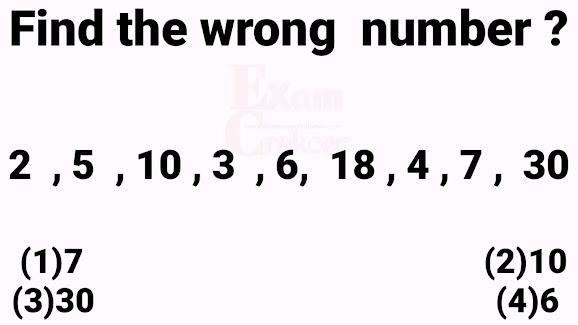## Ten most important problems of missing numbers in Reasoning Analogy part 2

Let us discuss series of missing numbers and missing terms and their  solutions in reasoning analogy . These questions are very very important for upcoming competitive exams like SSC CGL ,SSC CHSL and RRB NTPC Etc

## Problem # 1

#### This series consists of two alternate series 1st series consist of number 1,3, 5 and 7 and second series consist of  the series 2 ,5 ,8,?

So in 1st series there is difference of 2, so next number in the series must be 2 greater than 7 that is 9 .
In the 2nd series  we observed a difference of 3 show the next number must be 11 that is 3 greater than 8 the last term therefore the last two terms of the series 11 and 9

## This series is also the combination of two series one with the number  2, 48 ,16 ,32 and second series consist of these numbers 6, 9 13 ,18 , ? . So ? Will be filled out using 2nd series not from 1st series.

Observing 2nd series carefully ,In  second series numbers are increased by  3 ,4 and 5 ,so next Increment should be of 6. This implies next number should be  24 with an increment of 6.

## Problem # 5

#### Every number in this series is the sum of its two  preceeding number plus 3.

14  = ( 7 + 4 ) + 3
24 = ( 14 + 7 ) + 3
41 = ( 24  +14 ) + 3
?   = ( 41 + 24 ) + 3 = 65+3 = 68

## Problem # 6#### As third  number 10 is the product of 2 and 5, sixth number  18 is the product of fourth (3) and fifth (6 ) numbers .

So ninth number of the series  must be the product of seventh and  eighth numbers. 4 × 7 = 28 instead of 30

## Problem # 7

Here second  number is the sum of the digits of first number the forth number is the sum of digits of third number similarly every even positioned number is  the sum of its preceding number's digits question  mark will be replaced by the sum of 5 + 3 that is 8

## Problem # 8

Writing 3 = 2² - 1

#### Writing 24 = 5² - 1

Show next number should be  6² - 1 ( square minus 1 ) that is 35

## Problem # 9

Every number in this series is the double of its preceding number .So double of 1 is   2 , double of 2 is 4 ,double of 4 should be 8 ( instead of 7 ) , double of 8 is 16 and double of 16 should be 32 .  So 7 is the wrong number in this series.

## FINAL WORDS

Thanks for watching and responding to this post of reasoning based on mathematics and number sequence puzzle solver.

## Appeal

If you are a mathematician Don't forget to visit my Mathematics You tube channel ,Mathematics Website and Mathematics Facebook Page , whose links are given below

Also watch this video

Share: